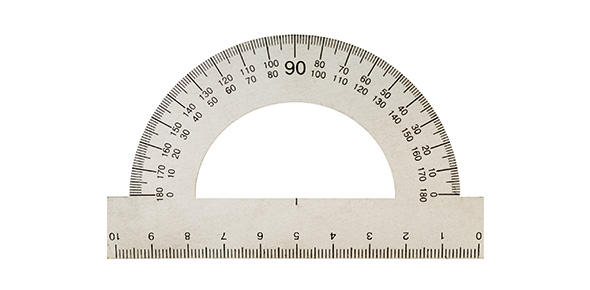# Giibilisco - Measuring Devices

18 Questions | Total Attempts: 1183Settings• 1.
The attraction or repulsion between two electrically charged objects is called
• A.

Electromagnetic deflection.

• B.

Electrostatic force.

• C.

Magnetic force.

• D.

Electroscopic force.

• 2.
The change in the direction of a compass needle, when a current-carrying wire is brought near, is called
• A.

Electromagnetic deflection.

• B.

Electrostatic force.

• C.

Magnetic force.

• D.

Electroscopic force.

• 3.
Suppose a certain current in a galvanometer causes the compass needle to deflect by 20 degrees, and then this current is doubled while the polarity stays the same. The angle of the needle deflection will
• A.

Decrease.

• B.

Stay the same.

• C.

Increase.

• D.

Reverse direction.

• 4.
One important advantage of an electrostatic meter is the fact that
• A.

It measures very small currents.

• B.

It can handle large currents.

• C.

It can detect and indicate ac voltages as well as dc voltages.

• D.

It draws a large current from a power supply.

• 5.
A thermocouple
• A.

Gets warm when dc flows through it.

• B.

Is a thin, straight, special wire.

• C.

Generates dc when exposed to visible light.

• D.

Generates ac when heated.

• 6.
An important advantage of an electromagnet-type meter over a permanent-magnet meter is the fact that
• A.

The electromagnet meter costs much less.

• B.

The electromagnet meter need not be aligned with the earth’s magnetic field.

• C.

The permanent-magnet meter has a more sluggish coil.

• D.

The electromagnet meter is more rugged.

• 7.
Ammeter shunts are useful because
• A.

They increase meter sensitivity.

• B.

They make a meter more physically rugged.

• C.

They allow for measurement of large currents.

• D.

They prevent overheating of the meter movement.

• 8.
Voltmeters should generally have
• A.

High internal resistance.

• B.

Low internal resistance.

• C.

The greatest possible sensitivity.

• D.

The ability to withstand large currents.

• 9.
In order to measure the power-supply voltage that is applied to an electrical circuit, a voltmeter should be placed
• A.

In series with the circuit that works from the supply.

• B.

Between the negative pole of the supply and the circuit working from the supply.

• C.

Between the positive pole of the supply and the circuit working from the supply.

• D.

In parallel with the circuit that works from the supply.

• 10.
Which of the following will not normally cause a large error in an ohmmeter reading?
• A.

A small voltage between points under test

• B.

A slight change in switchable internal resistance

• C.

A small change in the resistance to be measured

• D.

A slight error in the range switch position

• 11.
The main advantage of a FETVM over a conventional voltmeter is the fact that the FETVM
• A.

Can measure lower voltages.

• B.

Draws less current from the circuit under test.

• C.

Can withstand higher voltages safely.

• D.

Is sensitive to ac voltage as well as to dc voltage.

• 12.
Which of the following is not a function of a fuse?
• A.

To ensure there is enough current available for an appliance to work right

• B.

To make it impossible to use appliances that are too large for a given circuit

• C.

To limit the amount of power that a device can draw from the electrical circuit

• D.

To make sure the current drawn by an appliance cannot exceed a certain limit

• 13.
A utility meter’s motor speed depends directly on
• A.

The number of ampere-hours being used at the time.

• B.

The number of watt-hours being used at the time.

• C.

The number of watts being used at the time.

• D.

The number of kilowatt-hours being used at the time.

• 14.
• A.

Voltage.

• B.

Power.

• C.

Current.

• D.

Energy.

• 15.
A typical frequency counter
• A.

• B.

Is accurate to six digits or more.

• C.

Works by indirectly measuring current.

• D.

Works by indirectly measuring voltage.

• 16.
A VU meter is never used to get a general indication of
• A.

Sound intensity.

• B.

Decibels.

• C.

Power in an audio amplifier.

• D.

Visible light intensity.

• 17.
The meter movement in an illumination meter directly measures
• A.

Current.

• B.

Voltage.

• C.

Power.

• D.

Energy.

• 18.
An oscilloscope cannot be used to indicate
• A.

Frequency.

• B.

Wave shape.

• C.

Energy.

• D.

Peak signal voltage.

Related TopicsBack to top